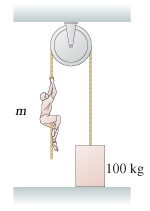# Problem: What is the tension in the rope of the figure? Assume that m = 64 kg.

###### FREE Expert Solution

From Newton's second law,

$\overline{){\mathbf{\Sigma }}{\mathbf{F}}{\mathbf{=}}{\mathbf{m}}{\mathbf{a}}}$

The tension in the rope supports a mass m.

For the mass m, tension acts upwards (positive) while weight acts downwards.

91% (384 ratings)###### Problem Details

What is the tension in the rope of the figure? Assume that m = 64 kg.